# Rotational Symmetry

An object has rotational symmetry if it looks the same after a turn of some size.

The number of times that the object matches up to itself during a full turn is called the order of the rotational symmetry.

For example, the star below has rotational symmetry of order 5. It matches up with itself 5 times as it passes through a full turn. I have added a small circle to one point of the star so that you can see it moving around as the star rotates:Do you see how the circle is back to where it started from in picture 5?

## Some Objects and Geometric Shapes with Rotational Symmetry

Order $2$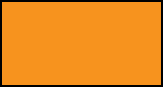Order $3$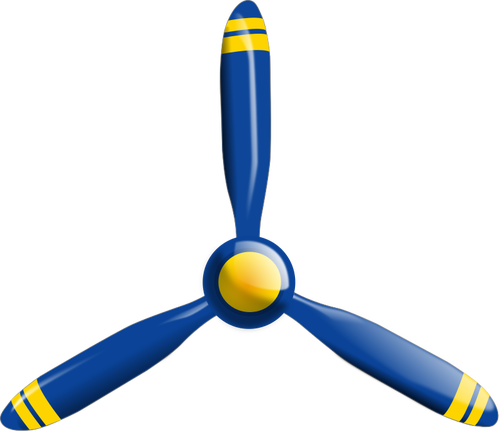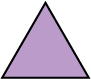Order $4$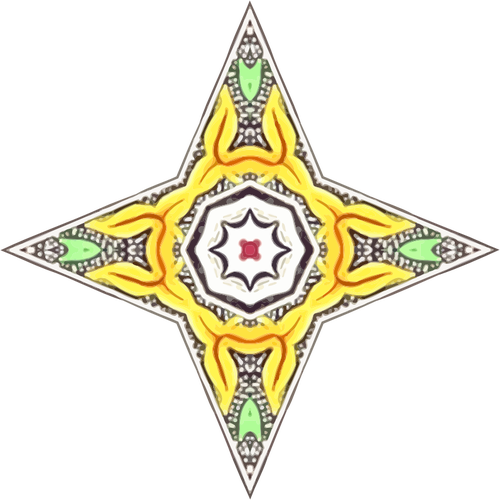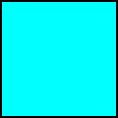'

Order $5$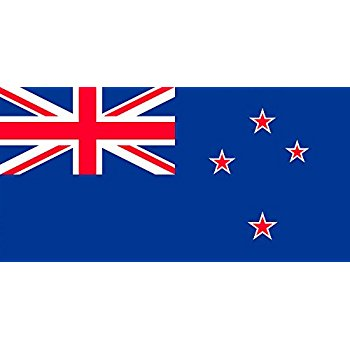The stars on the New Zealand flag have rotational symmetry of order $5$.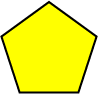Order $6$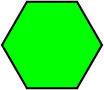Order $7$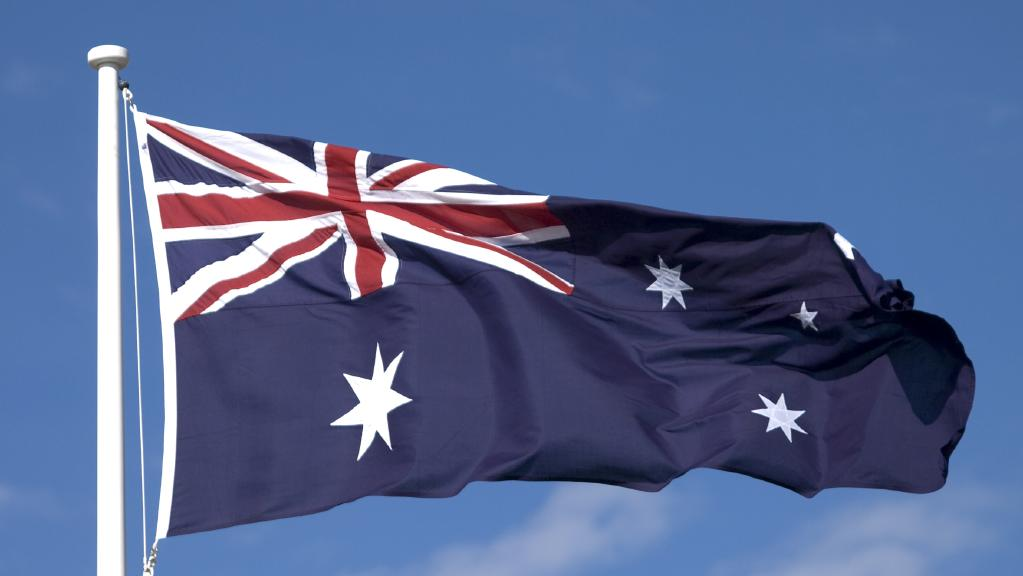All but one star on the Australian flag has rotational symmetry of order $7$.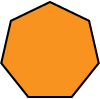Order $8$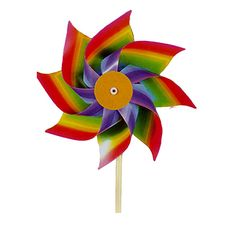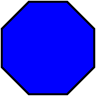Order $9$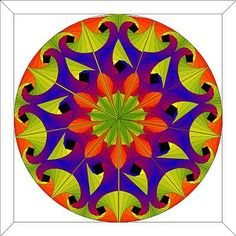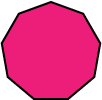Any regular polygon has rotational symmetry of order equal to its number of sides.

## Rotational Symmetry of Order 1?

Just think what rotational symmetry of order $1$ would mean. It wouldn't be very interesting, would it? It just says that the object matches up to itself after one whole turn. So, rotational symmetry of order one really means no rotational symmetry at all!

## Conclusion

Have a look around and see if you can spot any objects with rotational symmetry. Can you work out the orders?

Another name for rotational symmetry of order $2$ is point symmetry.

Have you thought about circles and rotational symmetry? I guess a circle has rotational symmetry of infinite order, because it will match up to itself after turning through any angle at all.

### Description

This mini book covers the core of Math for Foundation, Grade 1 and Grade 2 mathematics including

1. Numbers
3. Subtraction
4. Division
5. Algebra
6. Geometry
7. Data
8. Estimation
9. Probability/Chance
10. Measurement
11. Time
12. Money
13. and much more

This material is provided free of cost for Parent looking for some tricks for their Prekinder, Kinder, Prep, Year 1 and Year 2 children

### Learning Objectives

These lessons are for kids aged 4-8 with the core objective to expose their brains to concepts of addition, subtraction, division, algebra and much more.

Author: Subject Coach
You must be logged in as Student to ask a Question.